This mathematics ClipArt gallery offers 9 illustrations of circles that are often used in trigonometry. Included are images showing arcs, angles, chords, and radians.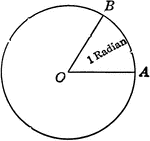Illustration of Circle with radian shown.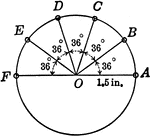### Circle With 36 degree Angles and Radius 1.5 in.

Circle with 36 degree angles marked. This diagram can be used with the following trig problem: Locate…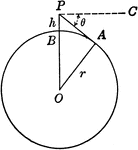### Circle With Center o and Radius r with point P

Circle modeling the earth. O is the center of the earth, r the radius of the earth, and h the height…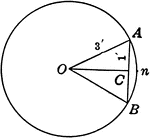### Circle With a Chord of 2 ft. and a Radius of 3 ft.

Circle with chord AB=2 ft. and radius OA = 3 ft.. Triangle AOC is a right triangle. Angle AOC=half angle…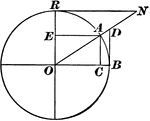### Versedsine and Coversedsine in Circle

The coversedsine is equal to 1 minus the sine. In this figure, AE is parallel to OB; hence, EO = AC…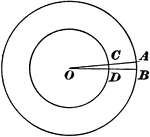### Concentric Circles With Angle of 1 °

"If AOB is an angle of 1 ° on the larger circle, it is also 1 ° on the smaller concentric circle,…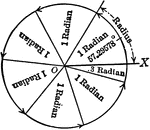Illustration showing that the radian is an angle that when placed at the center of a circle its sides…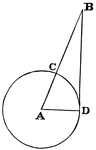### Circle with Secant

A secant is "a line which cuts a figure in any way. Specifically, in trigonometry, a line from the center…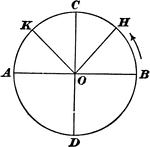### Arcs and Angles of a Trigonometric Circle

"In trigonometry, the arcs of circles are used to measure angles. All angles are supposed to have their…